# Z-Scores VIDEO

Calculating Z-Scores# Data Exploration Case Study: Credit Default

Exploratory data analysis is the main task of a Data Scientist with as much as 60% of their time being devoted to this task. As such, the majority of their time is spent on something that is rather boring compared to building models.This post will provide a simple example of how to analyze a dataset from the website called Kaggle. This dataset is looking at how is likely to default on their credit. The following steps will be conducted in this analysis.

1. Load the libraries and dataset
2. Deal with missing data
3. Some descriptive stats
4. Normality check
5. Model development

This is not an exhaustive analysis but rather a simple one for demonstration purposes. The dataset is available here

Here are some packages we will need

import pandas as pd
import matplotlib.pyplot as plt
import seaborn as sns
from scipy.stats import norm
from sklearn import tree
from scipy import stats
from sklearn import metrics

You can load the data with the code below

df_train=pd.read_csv('/application_train.csv')

You can examine what variables are available with the code below. This is not displayed here because it is rather long

df_train.columns
df_train.head()

Missing Data

I prefer to deal with missing data first because missing values can cause errors throughout the analysis if they are not dealt with immediately. The code below calculates the percentage of missing data in each column.

total=df_train.isnull().sum().sort_values(ascending=False)
percent=(df_train.isnull().sum()/df_train.isnull().count()).sort_values(ascending=False)
missing_data=pd.concat([total,percent],axis=1,keys=['Total','Percent'])

Total   Percent
COMMONAREA_MEDI           214865  0.698723
COMMONAREA_AVG            214865  0.698723
COMMONAREA_MODE           214865  0.698723
NONLIVINGAPARTMENTS_MODE  213514  0.694330
NONLIVINGAPARTMENTS_MEDI  213514  0.694330

Only the first five values are printed. You can see that some variables have a large amount of missing data. As such, they are probably worthless for inclusion in additional analysis. The code below removes all variables with any missing data.

pct_null = df_train.isnull().sum() / len(df_train)
missing_features = pct_null[pct_null > 0.0].index
df_train.drop(missing_features, axis=1, inplace=True)

You can use the .head() function if you want to see how  many variables are left.

Data Description & Visualization

For demonstration purposes, we will print descriptive stats and make visualizations of a few of the variables that are remaining.

round(df_train['AMT_CREDIT'].describe())
Out:
count     307511.0
mean      599026.0
std       402491.0
min        45000.0
25%       270000.0
50%       513531.0
75%       808650.0
max      4050000.0

sns.distplot(df_train['AMT_CREDIT']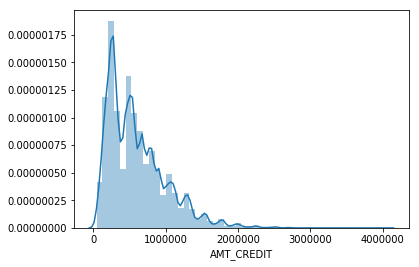round(df_train['AMT_INCOME_TOTAL'].describe())
Out:
count       307511.0
mean        168798.0
std         237123.0
min          25650.0
25%         112500.0
50%         147150.0
75%         202500.0
max      117000000.0
sns.distplot(df_train['AMT_INCOME_TOTAL']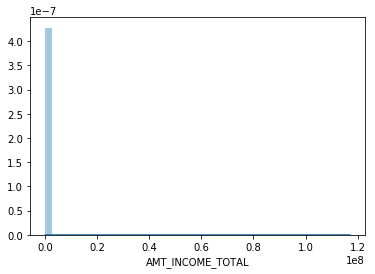I think you are getting the point. You can also look at categorical variables using the groupby() function.

We also need to address categorical variables in terms of creating dummy variables. This is so that we can develop a model in the future. Below is the code for dealing with all the categorical  variables and converting them to dummy variable’s

df_train.groupby('NAME_CONTRACT_TYPE').count()
dummy=pd.get_dummies(df_train['NAME_CONTRACT_TYPE'])
df_train=pd.concat([df_train,dummy],axis=1)
df_train=df_train.drop(['NAME_CONTRACT_TYPE'],axis=1)

df_train.groupby('CODE_GENDER').count()
dummy=pd.get_dummies(df_train['CODE_GENDER'])
df_train=pd.concat([df_train,dummy],axis=1)
df_train=df_train.drop(['CODE_GENDER'],axis=1)

df_train.groupby('FLAG_OWN_CAR').count()
dummy=pd.get_dummies(df_train['FLAG_OWN_CAR'])
df_train=pd.concat([df_train,dummy],axis=1)
df_train=df_train.drop(['FLAG_OWN_CAR'],axis=1)

df_train.groupby('FLAG_OWN_REALTY').count()
dummy=pd.get_dummies(df_train['FLAG_OWN_REALTY'])
df_train=pd.concat([df_train,dummy],axis=1)
df_train=df_train.drop(['FLAG_OWN_REALTY'],axis=1)

df_train.groupby('NAME_INCOME_TYPE').count()
dummy=pd.get_dummies(df_train['NAME_INCOME_TYPE'])
df_train=pd.concat([df_train,dummy],axis=1)
df_train=df_train.drop(['NAME_INCOME_TYPE'],axis=1)

df_train.groupby('NAME_EDUCATION_TYPE').count()
dummy=pd.get_dummies(df_train['NAME_EDUCATION_TYPE'])
df_train=pd.concat([df_train,dummy],axis=1)
df_train=df_train.drop(['NAME_EDUCATION_TYPE'],axis=1)

df_train.groupby('NAME_FAMILY_STATUS').count()
dummy=pd.get_dummies(df_train['NAME_FAMILY_STATUS'])
df_train=pd.concat([df_train,dummy],axis=1)
df_train=df_train.drop(['NAME_FAMILY_STATUS'],axis=1)

df_train.groupby('NAME_HOUSING_TYPE').count()
dummy=pd.get_dummies(df_train['NAME_HOUSING_TYPE'])
df_train=pd.concat([df_train,dummy],axis=1)
df_train=df_train.drop(['NAME_HOUSING_TYPE'],axis=1)

df_train.groupby('ORGANIZATION_TYPE').count()
dummy=pd.get_dummies(df_train['ORGANIZATION_TYPE'])
df_train=pd.concat([df_train,dummy],axis=1)
df_train=df_train.drop(['ORGANIZATION_TYPE'],axis=1)

You have to be careful with this because now you have many variables that are not necessary. For every categorical variable you must remove at least one category in order for the model to work properly.  Below we did this manually.

df_train=df_train.drop(['Revolving loans','F','XNA','N','Y','SK_ID_CURR,''Student','Emergency','Lower secondary','Civil marriage','Municipal apartment'],axis=1)

Below are some boxplots with the target variable and other variables in the dataset.

f,ax=plt.subplots(figsize=(8,6))
fig=sns.boxplot(x=df_train['TARGET'],y=df_train['AMT_INCOME_TOTAL'])There is a clear outlier there. Below is another boxplot with a different variable

f,ax=plt.subplots(figsize=(8,6))
fig=sns.boxplot(x=df_train['TARGET'],y=df_train['CNT_CHILDREN'])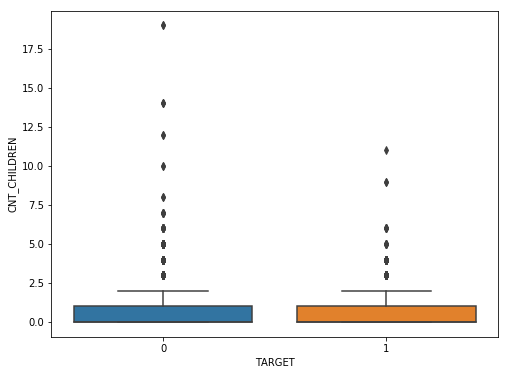It appears several people have more than 10 children. This is probably a typo.

Below is a correlation matrix using a heatmap technique

corrmat=df_train.corr()
f,ax=plt.subplots(figsize=(12,9))
sns.heatmap(corrmat,vmax=.8,square=True)The heatmap is nice but it is hard to really appreciate what is happening. The code below will sort the correlations from least to strongest, so we can remove high correlations.

c = df_train.corr().abs()

s = c.unstack()
so = s.sort_values(kind="quicksort")

FLAG_DOCUMENT_12 FLAG_MOBIL 0.000005
FLAG_MOBIL FLAG_DOCUMENT_12 0.000005
Unknown FLAG_MOBIL 0.000005
FLAG_MOBIL Unknown 0.000005
Cash loans FLAG_DOCUMENT_14 0.000005

The list is to long to show here but the following variables were removed for having a high correlation with other variables.

df_train=df_train.drop(['WEEKDAY_APPR_PROCESS_START','FLAG_EMP_PHONE','REG_CITY_NOT_WORK_CITY','REGION_RATING_CLIENT','REG_REGION_NOT_WORK_REGION'],axis=1)

Below we check a few variables for homoscedasticity, linearity, and normality  using plots and histograms

sns.distplot(df_train['AMT_INCOME_TOTAL'],fit=norm)
fig=plt.figure()
res=stats.probplot(df_train['AMT_INCOME_TOTAL'],plot=plt)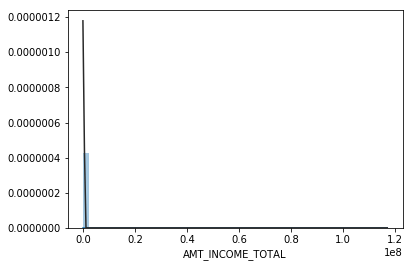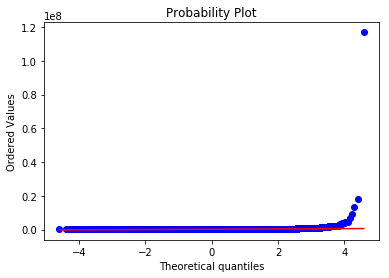This is not normal

sns.distplot(df_train['AMT_CREDIT'],fit=norm)
fig=plt.figure()
res=stats.probplot(df_train['AMT_CREDIT'],plot=plt)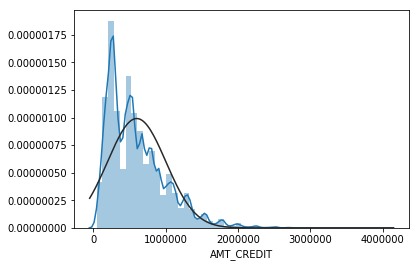This is not normal either. We could do transformations, or we can make a non-linear model instead.

Model Development

Now comes the easy part. We will make a decision tree using only some variables to predict the target. In the code below we make are X and y dataset.

X=df_train[['Cash loans','DAYS_EMPLOYED','AMT_CREDIT','AMT_INCOME_TOTAL','CNT_CHILDREN','REGION_POPULATION_RELATIVE']]
y=df_train['TARGET']

The code below fits are model and makes the predictions

clf=tree.DecisionTreeClassifier(min_samples_split=20)
clf=clf.fit(X,y)
y_pred=clf.predict(X)

Below is the confusion matrix followed by the accuracy

print (pd.crosstab(y_pred,df_train['TARGET']))
TARGET       0      1
row_0
0       280873  18493
1         1813   6332
accuracy_score(y_pred,df_train['TARGET'])
Out: 0.933966589813047

Lastly, we can look at the precision, recall, and f1 score

print(metrics.classification_report(y_pred,df_train['TARGET']))
precision    recall  f1-score   support

0       0.99      0.94      0.97    299366
1       0.26      0.78      0.38      8145

micro avg       0.93      0.93      0.93    307511
macro avg       0.62      0.86      0.67    307511
weighted avg       0.97      0.93      0.95    307511

This model looks rather good in terms of accuracy of the training set. It actually impressive that we could use so few variables from such a large dataset and achieve such a high degree of accuracy.

Conclusion

Data exploration and analysis is the primary task of a data scientist.  This post was just an example of how this can be approached. Of course, there are many other creative ways to do this but the simplistic nature of this analysis yielded strong results

# Confidence Intervals for Proportions VIDEO

Calculating Confidence Intervals for Proportions# Hierarchical Regression in R

In this post, we will learn how to conduct a hierarchical regression analysis in R. Hierarchical regression analysis is used in situation in which you want to see if adding additional variables to your model will significantly change the r2 when accounting for the other variables in the model. This approach is a model comparison approach and not necessarily a statistical one.We are going to use the “Carseats” dataset from the ISLR package. Our goal will be to predict total sales using the following independent variables in three different models.

model 1 = intercept only
model 2 = Sales~Urban + US + ShelveLoc
model 3 = Sales~Urban + US + ShelveLoc + price + income
model 4 = Sales~Urban + US + ShelveLoc + price + income + Advertising

Often the primary goal with hierarchical regression is to show that the addition of a new variable builds or improves upon a previous model in a statistically significant way. For example, if a previous model was able to predict the total sales of an object using three variables you may want to see if a new additional variable you have in mind may improve model performance. Another way to see this is in the following research question

Is a model that explains the total sales of an object with Urban location, US location, shelf location, price, income and advertising cost as independent variables superior in terms of R2 compared to a model that explains total sales with Urban location, US location, shelf location, price and income as independent variables?

In this complex research question we essentially want to know if adding advertising cost will improve the model significantly in terms of the r square. The formal steps that we will following to complete this analysis is as follows.

1. Build sequential (nested) regression models by adding variables at each step.
2. Run ANOVAs in order to compute the R2
3. Compute difference in sum of squares for each step
1. Check F-statistics and p-values for the SS differences.
4. Compare sum of squares between models from ANOVA results.
5. Compute increase in R2 from sum of square difference
6. Run regression to obtain the coefficients for each independent variable.

We will now begin our analysis. Below is some initial code

library(ISLR)
data("Carseats")

# Model Development

We now need to create our models. Model 1 will not have any variables in it and will be created for the purpose of obtaining the total sum of squares. Model 2 will include demographic variables. Model 3 will contain the initial model with the continuous independent variables. Lastly, model 4 will contain all the information of the previous models with the addition of the continuous independent variable of advertising cost. Below is the code.

model1 = lm(Sales~1,Carseats)
model2=lm(Sales~Urban + US + ShelveLoc,Carseats)
model3=lm(Sales~Urban + US + ShelveLoc + Price + Income,Carseats)
model4=lm(Sales~Urban + US + ShelveLoc + Price + Income + Advertising,Carseats)

We can now turn to the ANOVA analysis for model comparison #ANOVA Calculation We will use the anova() function to calculate the total sum of square for model 0. This will serve as a baseline for the other models for calculating r square

anova(model1,model2,model3,model4)
## Analysis of Variance Table
##
## Model 1: Sales ~ 1
## Model 2: Sales ~ Urban + US + ShelveLoc
## Model 3: Sales ~ Urban + US + ShelveLoc + Price + Income
## Model 4: Sales ~ Urban + US + ShelveLoc + Price + Income + Advertising
##   Res.Df    RSS Df Sum of Sq       F    Pr(>F)
## 1    399 3182.3
## 2    395 2105.4  4   1076.89  89.165 < 2.2e-16 ***
## 3    393 1299.6  2    805.83 133.443 < 2.2e-16 ***
## 4    392 1183.6  1    115.96  38.406 1.456e-09 ***
## ---
## Signif. codes:  0 '***' 0.001 '**' 0.01 '*' 0.05 '.' 0.1 ' ' 1

For now, we are only focusing on the residual sum of squares. Here is a basic summary of what we know as we compare the models.

model 1 = sum of squares = 3182.3
model 2 = sum of squares = 2105.4 (with demographic variables of Urban, US, and ShelveLoc)
model 3 = sum of squares = 1299.6 (add price and income)

Each model is statistical significant which means adding each variable lead to some improvement.

By adding price and income to the model we were able to improve the model in a statistically significant way. The r squared increased by .25 below is how this was calculated.

2105.4-1299.6 #SS of Model 2 - Model 3
##  805.8
805.8/ 3182.3 #SS difference of Model 2 and Model 3 divided by total sum of sqaure ie model 1
##  0.2532131

When we add Advertising to the model the r square increases by .03. The calculation is below

1299.6-1183.6 #SS of Model 3 - Model 4
##  116
116/ 3182.3 #SS difference of Model 3 and Model 4 divided by total sum of sqaure ie model 1
##  0.03645162

# Coefficients and R Square

We will now look at a summary of each model using the summary() function.

summary(model2)
##
## Call:
## lm(formula = Sales ~ Urban + US + ShelveLoc, data = Carseats)
##
## Residuals:
##    Min     1Q Median     3Q    Max
## -6.713 -1.634 -0.019  1.738  5.823
##
## Coefficients:
##                 Estimate Std. Error t value Pr(>|t|)
## (Intercept)       4.8966     0.3398  14.411  < 2e-16 ***
## UrbanYes          0.0999     0.2543   0.393   0.6947
## USYes             0.8506     0.2424   3.510   0.0005 ***
## ShelveLocGood     4.6400     0.3453  13.438  < 2e-16 ***
## ShelveLocMedium   1.8168     0.2834   6.410 4.14e-10 ***
## ---
## Signif. codes:  0 '***' 0.001 '**' 0.01 '*' 0.05 '.' 0.1 ' ' 1
##
## Residual standard error: 2.309 on 395 degrees of freedom
## Multiple R-squared:  0.3384, Adjusted R-squared:  0.3317
## F-statistic: 50.51 on 4 and 395 DF,  p-value: < 2.2e-16
summary(model3)
##
## Call:
## lm(formula = Sales ~ Urban + US + ShelveLoc + Price + Income,
##     data = Carseats)
##
## Residuals:
##     Min      1Q  Median      3Q     Max
## -4.9096 -1.2405 -0.0384  1.2754  4.7041
##
## Coefficients:
##                  Estimate Std. Error t value Pr(>|t|)
## (Intercept)     10.280690   0.561822  18.299  < 2e-16 ***
## UrbanYes         0.219106   0.200627   1.092    0.275
## USYes            0.928980   0.191956   4.840 1.87e-06 ***
## ShelveLocGood    4.911033   0.272685  18.010  < 2e-16 ***
## ShelveLocMedium  1.974874   0.223807   8.824  < 2e-16 ***
## Price           -0.057059   0.003868 -14.752  < 2e-16 ***
## Income           0.013753   0.003282   4.190 3.44e-05 ***
## ---
## Signif. codes:  0 '***' 0.001 '**' 0.01 '*' 0.05 '.' 0.1 ' ' 1
##
## Residual standard error: 1.818 on 393 degrees of freedom
## Multiple R-squared:  0.5916, Adjusted R-squared:  0.5854
## F-statistic: 94.89 on 6 and 393 DF,  p-value: < 2.2e-16
summary(model4)
##
## Call:
## lm(formula = Sales ~ Urban + US + ShelveLoc + Price + Income +
##
## Residuals:
##     Min      1Q  Median      3Q     Max
## -5.2199 -1.1703  0.0225  1.0826  4.1124
##
## Coefficients:
##                  Estimate Std. Error t value Pr(>|t|)
## (Intercept)     10.299180   0.536862  19.184  < 2e-16 ***
## UrbanYes         0.198846   0.191739   1.037    0.300
## USYes           -0.128868   0.250564  -0.514    0.607
## ShelveLocGood    4.859041   0.260701  18.638  < 2e-16 ***
## ShelveLocMedium  1.906622   0.214144   8.903  < 2e-16 ***
## Price           -0.057163   0.003696 -15.467  < 2e-16 ***
## Income           0.013750   0.003136   4.384 1.50e-05 ***
## Advertising      0.111351   0.017968   6.197 1.46e-09 ***
## ---
## Signif. codes:  0 '***' 0.001 '**' 0.01 '*' 0.05 '.' 0.1 ' ' 1
##
## Residual standard error: 1.738 on 392 degrees of freedom
## Multiple R-squared:  0.6281, Adjusted R-squared:  0.6214
## F-statistic: 94.56 on 7 and 392 DF,  p-value: < 2.2e-16

You can see for yourself the change in the r square. From model 2 to model 3 there is a 26 point increase in r square just as we calculated manually. From model 3 to model 4 there is a 3 point increase in r square. The purpose of the anova() analysis was determined if the significance of the change meet a statistical criterion, The lm() function reports a change but not the significance of it.

# Conclusion

Hierarchical regression is just another potential tool for the statistical researcher. It provides you with a way to develop several models and compare the results based on any potential improvement in the r square.

# Confidence Intervals VIDEO

Calculating Confidence intervals

# Standard Deviation VIDEO

Calculating standard deviation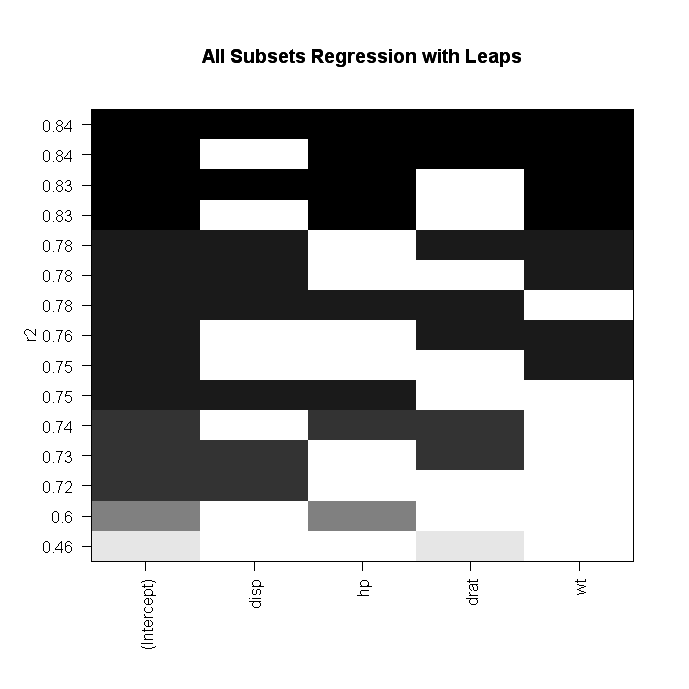# Subset Selection Regression

There are many different ways in which the variables of a regression model can be selected. In this post, we look at several common ways in which to selectvariables or features for a regression model. In particular, we look at the following.

• Best subset regression
• Stepwise selection

Best Subset Regression

Best subset regression fits a regression model for every possible combination of variables. The “best” model can be selected based on such criteria as the adjusted r-square, BIC (Bayesian Information Criteria), etc.

The primary drawback to best subset regression is that it becomes impossible to compute the results when you have a large number of variables. Generally, when the number of variables exceeds 40 best subset regression becomes too difficult to calculate.

Stepwise Selection

Stepwise selection involves adding or taking away one variable at a time from a regression model. There are two forms of stepwise selection and they are forward and backward selection.

In forward selection, the computer starts with a null model ( a model that calculates the mean) and adds one variable at a time to the model. The variable chosen is the one the provides the best improvement to the model fit. This process reduces greatly the number of models that need to be fitted in comparison to best subset regression.

Backward selection starts the full model and removes one variable at a time based on which variable improves the model fit the most. The main problem with either forward or backward selection is that the best model may not always be selected in this process. In addition, backward selection must have a sample size that is larger than the number of variables.

Deciding Which to Choose

Best subset regression is perhaps most appropriate when you have a small number of variables to develop a model with, such as less than 40. When the number of variables grows forward or backward selection are appropriate. If the sample size is small forward selection may be a better choice. However, if the sample size is large as in the number of examples is greater than the number of variables it is now possible to use backward selection.

Conclusion

The examples here are some of the most basic ways to develop a regression model. However, these are not the only ways in which this can be done. What these examples provide is an introduction to regression model development. In addition, these models provide some sort of criteria for the addition or removal of a variable based on statistics rather than intuition.

# Linear Regression vs Bayesian Regression

In this post, we are going to look at Bayesian regression. In particular, we will compare the results of ordinary least squares regression with Bayesian regression.

Bayesian Statistics

Bayesian statistics involves the use of probabilities rather than frequencieswhen addressing uncertainty. This allows you to determine the distribution of the model parameters and not only the values. This is done through averaging over the model parameters through marginalizing the joint probability distribution.

Linear Regression

We will now develop our two models. The first model will be a normal regression and the second a Bayesian model. We will be looking at factors that affect the tax rate of homes in the “Hedonic” dataset in the “Ecdat” package. We will load our packages and partition our data. Below is some initial code

library(ISLR);library(caret);library(arm);library(Ecdat);library(gridExtra)
data("Hedonic")
inTrain<-createDataPartition(y=Hedonic$tax,p=0.7, list=FALSE) trainingset <- Hedonic[inTrain, ] testingset <- Hedonic[-inTrain, ] str(Hedonic) ## 'data.frame': 506 obs. of 15 variables: ##$ mv     : num  10.09 9.98 10.45 10.42 10.5 ...
##  $crim : num 0.00632 0.02731 0.0273 0.03237 0.06905 ... ##$ zn     : num  18 0 0 0 0 0 12.5 12.5 12.5 12.5 ...
##  $indus : num 2.31 7.07 7.07 2.18 2.18 ... ##$ chas   : Factor w/ 2 levels "no","yes": 1 1 1 1 1 1 1 1 1 1 ...
##  $nox : num 28.9 22 22 21 21 ... ##$ rm     : num  43.2 41.2 51.6 49 51.1 ...
##  $age : num 65.2 78.9 61.1 45.8 54.2 ... ##$ dis    : num  1.41 1.6 1.6 1.8 1.8 ...
##  $rad : num 0 0.693 0.693 1.099 1.099 ... ##$ tax    : int  296 242 242 222 222 222 311 311 311 311 ...
##  $ptratio: num 15.3 17.8 17.8 18.7 18.7 ... ##$ blacks : num  0.397 0.397 0.393 0.395 0.397 ...
##  $lstat : num -3 -2.39 -3.21 -3.53 -2.93 ... ##$ townid : int  1 2 2 3 3 3 4 4 4 4 ...

We will now create our regression model

ols.reg<-lm(tax~.,trainingset)
summary(ols.reg)
##
## Call:
## lm(formula = tax ~ ., data = trainingset)
##
## Residuals:
##      Min       1Q   Median       3Q      Max
## -180.898  -35.276    2.731   33.574  200.308
##
## Coefficients:
##             Estimate Std. Error t value Pr(>|t|)
## (Intercept) 305.1928   192.3024   1.587  0.11343
## mv          -41.8746    18.8490  -2.222  0.02697 *
## crim          0.3068     0.6068   0.506  0.61339
## zn            1.3278     0.2006   6.618 1.42e-10 ***
## indus         7.0685     0.8786   8.045 1.44e-14 ***
## chasyes     -17.0506    15.1883  -1.123  0.26239
## nox           0.7005     0.4797   1.460  0.14518
## rm           -0.1840     0.5875  -0.313  0.75431
## age           0.3054     0.2265   1.349  0.17831
## dis          -7.4484    14.4654  -0.515  0.60695
## rad          98.9580     6.0964  16.232  < 2e-16 ***
## ptratio       6.8961     2.1657   3.184  0.00158 **
## blacks      -29.6389    45.0043  -0.659  0.51061
## lstat       -18.6637    12.4674  -1.497  0.13532
## townid        1.1142     0.1649   6.758 6.07e-11 ***
## ---
## Signif. codes:  0 '***' 0.001 '**' 0.01 '*' 0.05 '.' 0.1 ' ' 1
##
## Residual standard error: 63.72 on 341 degrees of freedom
## Multiple R-squared:  0.8653, Adjusted R-squared:  0.8597
## F-statistic: 156.4 on 14 and 341 DF,  p-value: < 2.2e-16

The model does a reasonable job. Next, we will do our prediction and compare the results with the test set using correlation, summary statistics, and the mean absolute error. In the code below, we use the “predict.lm” function and include the arguments “interval” for the prediction as well as “se.fit” for the standard error

ols.regTest<-predict.lm(ols.reg,testingset,interval = 'prediction',se.fit = T)

Below is the code for the correlation, summary stats, and mean absolute error. For MAE, we need to create a function.

cor(testingset$tax,ols.regTest$fit[,1])
##  0.9313795
summary(ols.regTest$fit[,1]) ## Min. 1st Qu. Median Mean 3rd Qu. Max. ## 144.7 288.3 347.6 399.4 518.4 684.1 summary(trainingset$tax)
##    Min. 1st Qu.  Median    Mean 3rd Qu.    Max.
##   188.0   279.0   330.0   410.4   666.0   711.0
MAE<-function(actual, predicted){
mean(abs(actual-predicted))
}
MAE(ols.regTest$fit[,1], testingset$tax)
##  41.07212

The correlation is excellent. The summary stats are similar and the error is not unreasonable. Below is a plot of the actual and predicted values

We now need to combine some data into one dataframe. In particular, we need the following actual dependent variable results predicted dependent variable results The upper confidence value of the prediction THe lower confidence value of the prediction

The code is below

yout.ols <- as.data.frame(cbind(testingset$tax,ols.regTest$fit))
ols.upr <- yout.ols$upr ols.lwr <- yout.ols$lwr

We can now plot this

p.ols <- ggplot(data = yout.ols, aes(x = testingset$tax, y = ols.regTest$fit[,1])) + geom_point() + ggtitle("Ordinary Regression") + labs(x = "Actual", y = "Predicted")
p.ols + geom_errorbar(ymin = ols.lwr, ymax = ols.upr)You can see the strong linear relationship. However, the confidence intervals are rather wide. Let’s see how Bayes does.

Bayes Regression

Bayes regression uses the “bayesglm” function from the “arm” package. We need to set the family to “gaussian” and the link to “identity”. In addition, we have to set the “prior.df” (prior degrees of freedom) to infinity as this indicates we want a normal distribution

bayes.reg<-bayesglm(tax~.,family=gaussian(link=identity),trainingset,prior.df = Inf)
bayes.regTest<-predict.glm(bayes.reg,newdata = testingset,se.fit = T)
cor(testingset$tax,bayes.regTest$fit)
##  0.9313793
summary(bayes.regTest$fit) ## Min. 1st Qu. Median Mean 3rd Qu. Max. ## 144.7 288.3 347.5 399.4 518.4 684.1 summary(trainingset$tax)
##    Min. 1st Qu.  Median    Mean 3rd Qu.    Max.
##   188.0   279.0   330.0   410.4   666.0   711.0
MAE(bayes.regTest$fit, testingset$tax)
##  41.07352

The numbers are essentially the same. This leads to the question of what is the benefit of Bayesian regression? The answer is in the confidence intervals. Next, we will calculate the confidence intervals for the Bayesian model.

yout.bayes <- as.data.frame(cbind(testingset$tax,bayes.regTest$fit))
names(yout.bayes) <- c("tax", "fit")
critval <- 1.96 #approx for 95% CI
bayes.upr <- bayes.regTest$fit + critval * bayes.regTest$se.fit
bayes.lwr <- bayes.regTest$fit - critval * bayes.regTest$se.fit

We now create our Bayesian regression plot.

p.bayes <- ggplot(data = yout.bayes, aes(x = yout.bayes$tax, y = yout.bayes$fit)) + geom_point() + ggtitle("Bayesian Regression Prediction") + labs(x = "Actual", y = "Predicted")

Lastly, we display both plots as a comparison.

ols.plot <-  p.ols + geom_errorbar(ymin = ols.lwr, ymax = ols.upr)
bayes.plot <-  p.bayes + geom_errorbar(ymin = bayes.lwr, ymax = bayes.upr)
grid.arrange(ols.plot,bayes.plot,ncol=2)As you can see, the Bayesian approach gives much more compact confidence intervals. This is because the Bayesian approach a distribution of parameters is calculated from a posterior distribution. These values are then averaged to get the final prediction that appears on the plot. This reduces the variance and strengthens the confidence we can have in each individual example.

# Linear VS Quadratic Discriminant Analysis in R

In this post, we will look at linear discriminant analysis (LDA) and quadratic discriminant analysis (QDA). Discriminant analysis is used when the dependent variable is categorical. Another commonly used option is logistic regression but there are differences between logistic regression and discriminant analysis. Both LDA and QDA are used in situations in which there is a clear separationbetween the classes you want to predict. If the categories are fuzzier logistic regression is often the better choice.

For our example, we will use the “Mathlevel” dataset found in the “Ecdat” package. Our goal will be to predict the sex of a respondent based on SAT math score, major, foreign language proficiency, as well as the number of math, physic, and chemistry classes a respondent took. Below is some initial code to start our analysis.

library(MASS);library(Ecdat)
data("Mathlevel")

The first thing we need to do is clean up the data set. We have to remove any missing data in order to run our model. We will create a dataset called “math” that has the “Mathlevel” dataset but with the “NA”s removed use the “na.omit” function. After this, we need to set our seed for the purpose of reproducibility using the “set.seed” function. Lastly, we will split the data using the “sample” function using a 70/30 split. The training dataset will be called “math.train” and the testing dataset will be called “math.test”. Below is the code

math<-na.omit(Mathlevel)
set.seed(123)
math.ind<-sample(2,nrow(math),replace=T,prob = c(0.7,0.3))
math.train<-math[math.ind==1,]
math.test<-math[math.ind==2,]

Now we will make our model and it is called “lda.math” and it will include all available variables in the “math.train” dataset. Next, we will check the results by calling the model. Finally, we will examine the plot to see how our model is doing. Below is the code.

lda.math<-lda(sex~.,math.train)
lda.math
## Call:
## lda(sex ~ ., data = math.train)
##
## Prior probabilities of groups:
##      male    female
## 0.5986079 0.4013921
##
## Group means:
##        mathlevel.L mathlevel.Q mathlevel.C mathlevel^4 mathlevel^5
## male   -0.10767593  0.01141838 -0.05854724   0.2070778  0.05032544
## female -0.05571153  0.05360844 -0.08967303   0.2030860 -0.01072169
##        mathlevel^6      sat languageyes  majoreco  majoross   majorns
## male    -0.2214849 632.9457  0.07751938 0.3914729 0.1472868 0.1782946
## female  -0.2226767 613.6416  0.19653179 0.2601156 0.1907514 0.2485549
##          majorhum mathcourse physiccourse chemistcourse
## male   0.05426357   1.441860    0.7441860      1.046512
## female 0.07514451   1.421965    0.6531792      1.040462
##
## Coefficients of linear discriminants:
##                       LD1
## mathlevel.L    1.38456344
## mathlevel.Q    0.24285832
## mathlevel.C   -0.53326543
## mathlevel^4    0.11292817
## mathlevel^5   -1.24162715
## mathlevel^6   -0.06374548
## sat           -0.01043648
## languageyes    1.50558721
## majoreco      -0.54528930
## majoross       0.61129797
## majorns        0.41574298
## majorhum       0.33469586
## mathcourse    -0.07973960
## physiccourse  -0.53174168
## chemistcourse  0.16124610
plot(lda.math,type='both')Calling “lda.math” gives us the details of our model. It starts be indicating the prior probabilities of someone being male or female. Next is the means for each variable by sex. The last part is the coefficients of the linear discriminants. Each of these values is used to determine the probability that a particular example is male or female. This is similar to a regression equation.

The plot provides us with densities of the discriminant scores for males and then for females. The output indicates a problem. There is a great deal of overlap between male and females in the model. What this indicates is that there is a lot of misclassification going on as the two groups are not clearly separated. Furthermore, this means that logistic regression is probably a better choice for distinguishing between male and females. However, since this is for demonstrating purposes we will not worry about this.

We will now use the “predict” function on the training set data to see how well our model classifies the respondents by gender. We will then compare the prediction of the model with the actual classification. Below is the code.

math.lda.predict<-predict(lda.math)
math.train$lda<-math.lda.predict$class
table(math.train$lda,math.train$sex)
##
##          male female
##   male    219    100
##   female   39     73
mean(math.train$lda==math.train$sex)
##  0.6774942

As you can see, we have a lot of misclassification happening. A large amount of false negatives which is a lot of males being classified as female. The overall accuracy is only 59% which is not much better than chance.

We will now conduct the same analysis on the test data set. Below is the code.

lda.math.test<-predict(lda.math,math.test)
math.test$lda<-lda.math.test$class
table(math.test$lda,math.test$sex)
##
##          male female
##   male     92     43
##   female   23     20
mean(math.test$lda==math.test$sex)
##  0.6292135

As you can see the results are similar. To put it simply, our model is terrible. The main reason is that there is little distinction between males and females as shown in the plot. However, we can see if perhaps a quadratic discriminant analysis will do better

QDA allows for each class in the dependent variable to have its own covariance rather than a shared covariance as in LDA. This allows for quadratic terms in the development of the model. To complete a QDA we need to use the “qda” function from the “MASS” package. Below is the code for the training data set.

math.qda.fit<-qda(sex~.,math.train)
math.qda.predict<-predict(math.qda.fit)
math.train$qda<-math.qda.predict$class
table(math.train$qda,math.train$sex)
##
##          male female
##   male    215     84
##   female   43     89
mean(math.train$qda==math.train$sex)
##  0.7053364

You can see there is almost no difference. Below is the code for the test data.

math.qda.test<-predict(math.qda.fit,math.test)
math.test$qda<-math.qda.test$class
table(math.test$qda,math.test$sex)
##
##          male female
##   male     91     43
##   female   24     20
mean(math.test$qda==math.test$sex)
##  0.6235955

Still disappointing. However, in this post, we reviewed linear discriminant analysis as well as learned about the use of quadratic linear discriminant analysis. Both of these statistical tools are used for predicting categorical dependent variables. LDA assumes shared covariance in the dependent variable categories will QDA allows for each category in the dependent variable to have its own variance.

# Linear Discriminant Analysis in R

In this post we will look at an example of linear discriminant analysis (LDA). LDA is used to develop a statistical model that classifies examples in a dataset. In the example in this post, we will use the “Star” dataset from the “Ecdat” package. What we will do is try to predict the type of class the students learned in (regular, small, regular with aide) using their math scores, reading scores, and the teaching experience of the teacher. Below is the initial code

library(Ecdat)
library(MASS)
data(Star)

We first need to examine the data by using the “str” function

str(Star)
## 'data.frame':    5748 obs. of  8 variables:
##  $tmathssk: int 473 536 463 559 489 454 423 500 439 528 ... ##$ treadssk: int  447 450 439 448 447 431 395 451 478 455 ...
##  $classk : Factor w/ 3 levels "regular","small.class",..: 2 2 3 1 2 1 3 1 2 2 ... ##$ totexpk : int  7 21 0 16 5 8 17 3 11 10 ...
##  $sex : Factor w/ 2 levels "girl","boy": 1 1 2 2 2 2 1 1 1 1 ... ##$ freelunk: Factor w/ 2 levels "no","yes": 1 1 2 1 2 2 2 1 1 1 ...
##  $race : Factor w/ 3 levels "white","black",..: 1 2 2 1 1 1 2 1 2 1 ... ##$ schidkn : int  63 20 19 69 79 5 16 56 11 66 ...
##  - attr(*, "na.action")=Class 'omit'  Named int [1:5850] 1 4 6 7 8 9 10 15 16 17 ...
##   .. ..- attr(*, "names")= chr [1:5850] "1" "4" "6" "7" ...

We will use the following variables

• dependent variable = classk (class type)
• independent variable = tmathssk (Math score)
• independent variable = totexpk (Teaching experience)

We now need to examine the data visually by looking at histograms for our independent variables and a table for our dependent variable

hist(Star$tmathssk)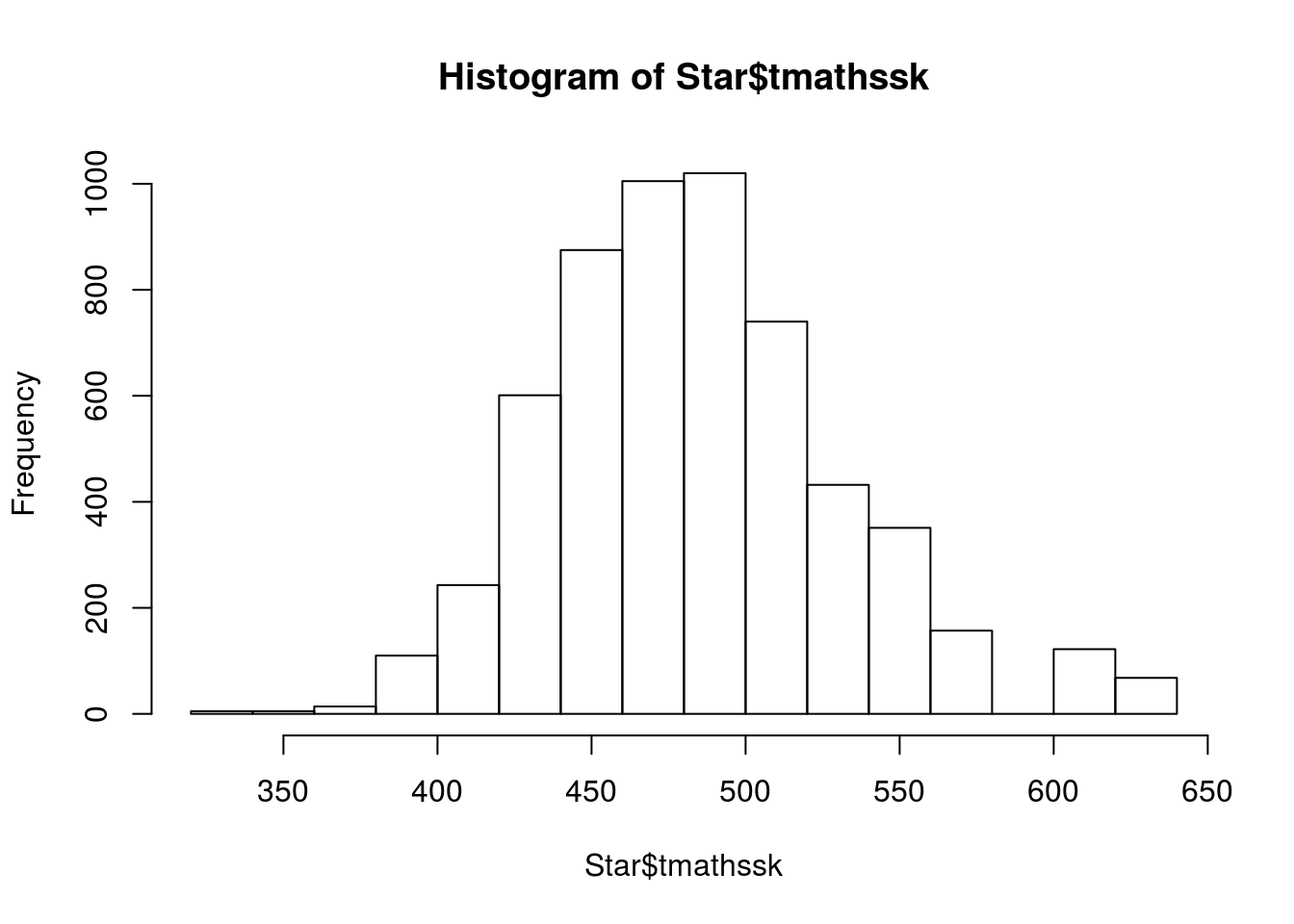hist(Star$treadssk)hist(Star$totexpk)prop.table(table(Star$classk))
##
##           regular       small.class regular.with.aide
##         0.3479471         0.3014962         0.3505567

The data mostly looks good. The results of the “prop.table” function will help us when we develop are training and testing datasets. The only problem is with the “totexpk” variable. IT is not anywhere near to be normally distributed. TO deal with this we will use the square root for teaching experience. Below is the code

star.sqrt<-Star
star.sqrt$totexpk.sqrt<-sqrt(star.sqrt$totexpk)
hist(sqrt(star.sqrt$totexpk))Much better. We now need to check the correlation among the variables as well and we will use the code below. cor.star<-data.frame(star.sqrt$tmathssk,star.sqrt$treadssk,star.sqrt$totexpk.sqrt)
cor(cor.star)
##                        star.sqrt.tmathssk star.sqrt.treadssk
## star.sqrt.tmathssk             1.00000000          0.7135489
## star.sqrt.totexpk.sqrt         0.08647957          0.1045353
##                        star.sqrt.totexpk.sqrt
## star.sqrt.tmathssk                 0.08647957
## star.sqrt.totexpk.sqrt             1.00000000

None of the correlations are too bad. We can now develop our model using linear discriminant analysis. First, we need to scale are scores because the test scores and the teaching experience are measured differently. Then, we need to divide our data into a train and test set as this will allow us to determine the accuracy of the model. Below is the code.

star.sqrt$tmathssk<-scale(star.sqrt$tmathssk)
star.sqrt$treadssk<-scale(star.sqrt$treadssk)
star.sqrt$totexpk.sqrt<-scale(star.sqrt$totexpk.sqrt)
train.star<-star.sqrt[1:4000,]
test.star<-star.sqrt[4001:5748,]

Now we develop our model. In the code before the “prior” argument indicates what we expect the probabilities to be. In our data the distribution of the the three class types is about the same which means that the apriori probability is 1/3 for each class type.

train.lda<-lda(classk~tmathssk+treadssk+totexpk.sqrt, data =
train.star,prior=c(1,1,1)/3)
train.lda
## Call:
## lda(classk ~ tmathssk + treadssk + totexpk.sqrt, data = train.star,
##     prior = c(1, 1, 1)/3)
##
## Prior probabilities of groups:
##           regular       small.class regular.with.aide
##         0.3333333         0.3333333         0.3333333
##
## Group means:
## regular           -0.04237438 -0.05258944  -0.05082862
## small.class        0.13465218  0.11021666  -0.02100859
## regular.with.aide -0.05129083 -0.01665593   0.09068835
##
## Coefficients of linear discriminants:
##                      LD1         LD2
## tmathssk      0.89656393 -0.04972956
## totexpk.sqrt -0.49061950  0.80051026
##
## Proportion of trace:
##    LD1    LD2
## 0.7261 0.2739

The printout is mostly readable. At the top is the actual code used to develop the model followed by the probabilities of each group. The next section shares the means of the groups. The coefficients of linear discriminants are the values used to classify each example. The coefficients are similar to regression coefficients. The computer places each example in both equations and probabilities are calculated. Whichever class has the highest probability is the winner. In addition, the higher the coefficient the more weight it has. For example, “tmathssk” is the most influential on LD1 with a coefficient of 0.89.

The proportion of trace is similar to principal component analysis

Now we will take the trained model and see how it does with the test set. We create a new model called “predict.lda” and use are “train.lda” model and the test data called “test.star”

predict.lda<-predict(train.lda,newdata = test.star)

We can use the “table” function to see how well are model has done. We can do this because we actually know what class our data is beforehand because we divided the dataset. What we need to do is compare this to what our model predicted. Therefore, we compare the “classk” variable of our “test.star” dataset with the “class” predicted by the “predict.lda” model.

table(test.star$classk,predict.lda$class)
##
##                     regular small.class regular.with.aide
##   regular               155         182               249
##   small.class           145         198               174
##   regular.with.aide     172         204               269

The results are pretty bad. For example, in the first row called “regular” we have 155 examples that were classified as “regular” and predicted as “regular” by the model. In rhe next column, 182 examples that were classified as “regular” but predicted as “small.class”, etc. To find out how well are model did you add together the examples across the diagonal from left to right and divide by the total number of examples. Below is the code

(155+198+269)/1748
##  0.3558352

Only 36% accurate, terrible but ok for a demonstration of linear discriminant analysis. Since we only have two-functions or two-dimensions we can plot our model.  Below I provide a visual of the first 50 examples classified by the predict.lda model.

plot(predict.lda$x[1:50]) text(predict.lda$x[1:50],as.character(predict.lda$class[1:50]),col=as.numeric(predict.lda$class[1:100]))
abline(h=0,col="blue")
abline(v=0,col="blue")The first function, which is the vertical line, doesn’t seem to discriminant anything as it off to the side and not separating any of the data. However, the second function, which is the horizontal one, does a good of dividing the “regular.with.aide” from the “small.class”. Yet, there are problems with distinguishing the class “regular” from either of the other two groups.  In order improve our model we need additional independent variables to help to distinguish the groups in the dependent variable.

# Generalized Additive Models in R

In this post, we will learn how to create a generalized additive model (GAM). GAMs are non-parametric generalized linear models. This means that linear predictor of the model uses smooth functions onthe predictor variables. As such, you do not need to specify the functional relationship between the response and continuous variables. This allows you to explore the data for potential relationships that can be more rigorously tested with other statistical models

In our example, we will use the “Auto” dataset from the “ISLR” package and use the variables “mpg”,“displacement”,“horsepower”, and “weight” to predict “acceleration”. We will also use the “mgcv” package. Below is some initial code to begin the analysis

library(mgcv)
library(ISLR)
data(Auto)

We will now make the model we want to understand the response of “acceleration” to the explanatory variables of “mpg”,“displacement”,“horsepower”, and “weight”. After setting the model we will examine the summary. Below is the code

model1<-gam(acceleration~s(mpg)+s(displacement)+s(horsepower)+s(weight),data=Auto)
summary(model1)
##
## Family: gaussian
##
## Formula:
## acceleration ~ s(mpg) + s(displacement) + s(horsepower) + s(weight)
##
## Parametric coefficients:
##             Estimate Std. Error t value Pr(>|t|)
## (Intercept) 15.54133    0.07205   215.7   <2e-16 ***
## ---
## Signif. codes:  0 '***' 0.001 '**' 0.01 '*' 0.05 '.' 0.1 ' ' 1
##
## Approximate significance of smooth terms:
##                   edf Ref.df      F  p-value
## s(mpg)          6.382  7.515  3.479  0.00101 **
## s(displacement) 1.000  1.000 36.055 4.35e-09 ***
## s(horsepower)   4.883  6.006 70.187  < 2e-16 ***
## s(weight)       3.785  4.800 41.135  < 2e-16 ***
## ---
## Signif. codes:  0 '***' 0.001 '**' 0.01 '*' 0.05 '.' 0.1 ' ' 1
##
## R-sq.(adj) =  0.733   Deviance explained = 74.4%
## GCV = 2.1276  Scale est. = 2.0351    n = 392

All of the explanatory variables are significant and the adjust r-squared is .73 which is excellent. edf stands for “effective degrees of freedom”. This modified version of the degree of freedoms is due to the smoothing process in the model. GCV stands for generalized cross-validation and this number is useful when comparing models. The model with the lowest number is the better model.

We can also examine the model visually by using the “plot” function. This will allow us to examine if the curvature fitted by the smoothing process was useful or not for each variable. Below is the code.

plot(model1)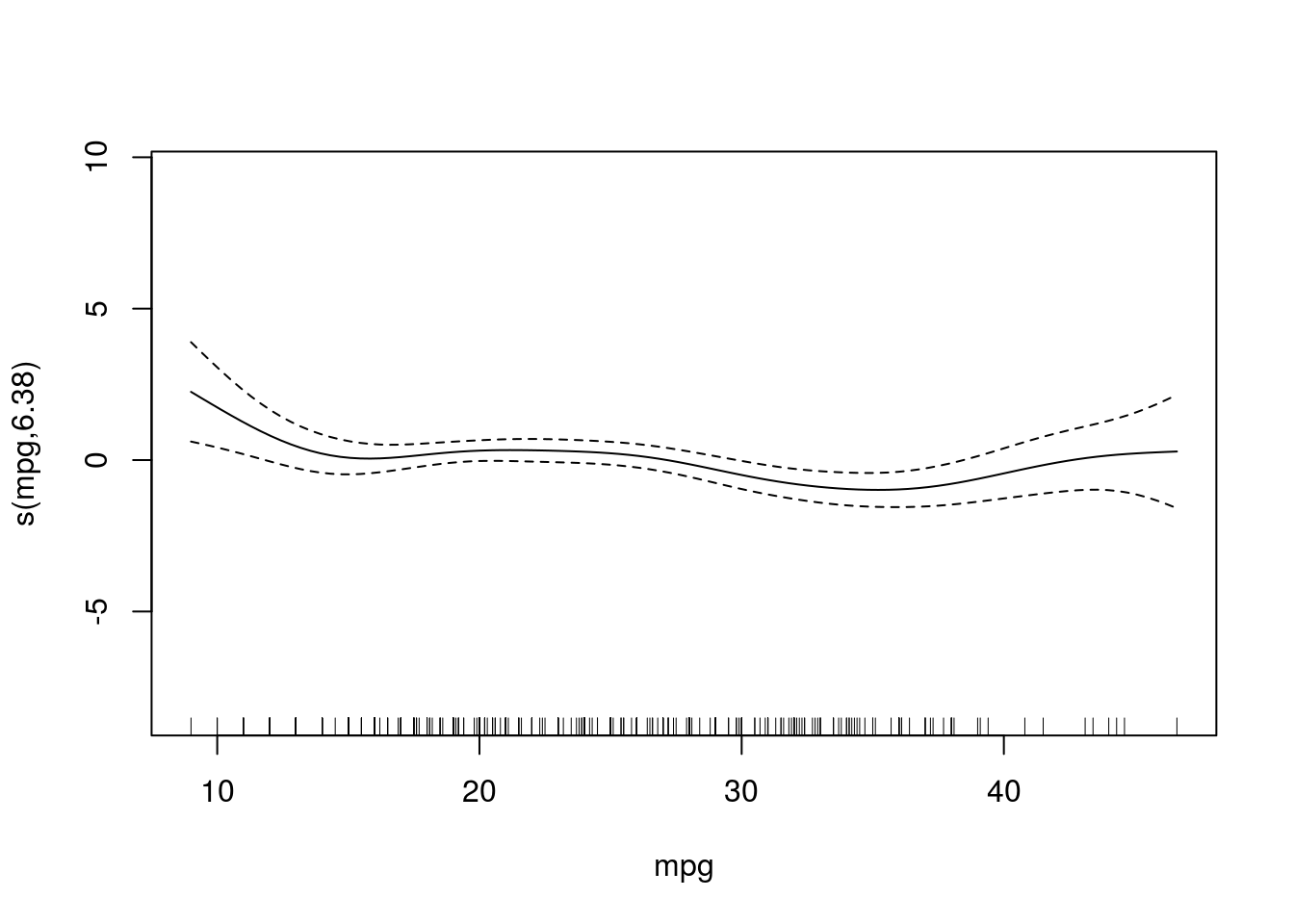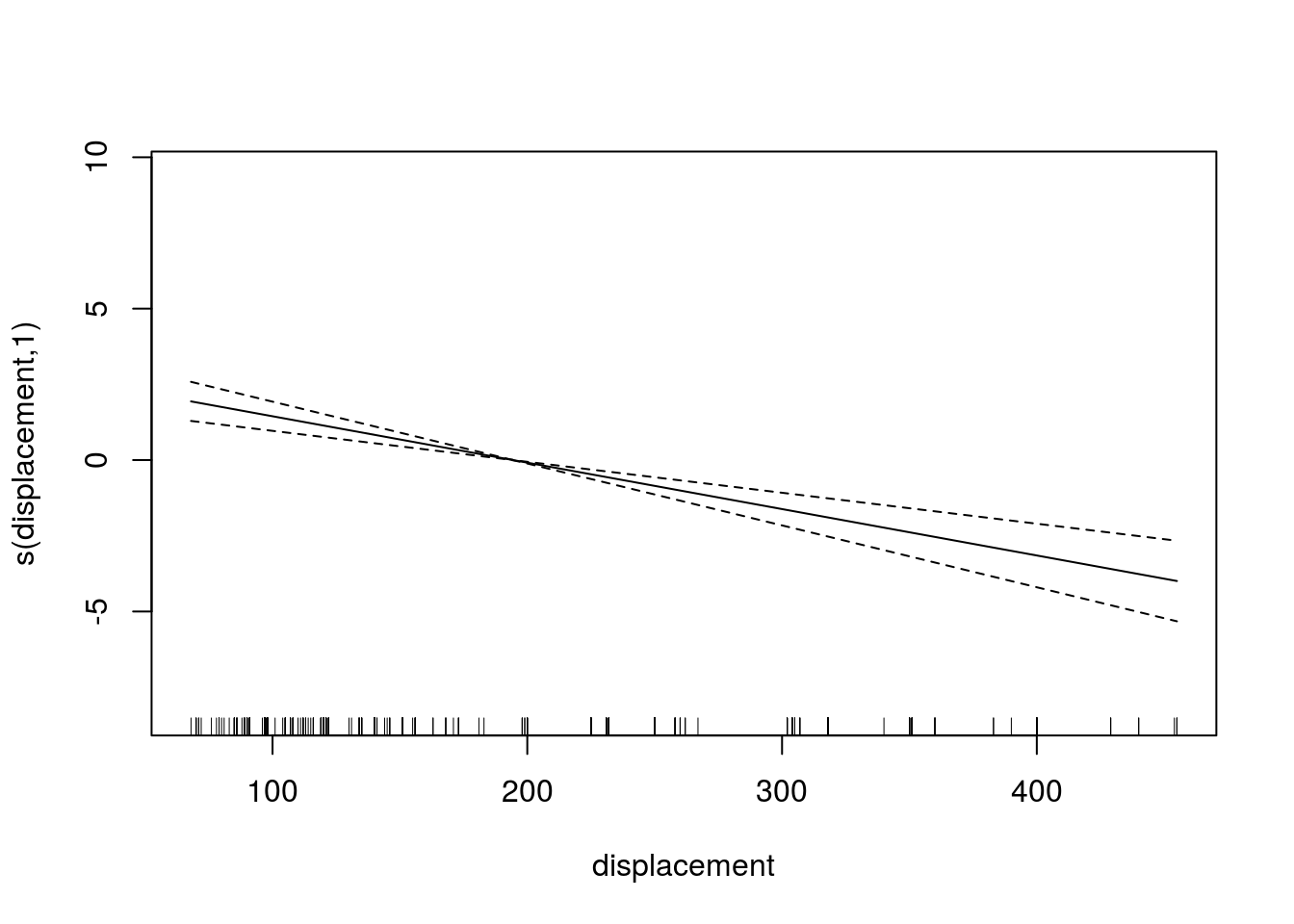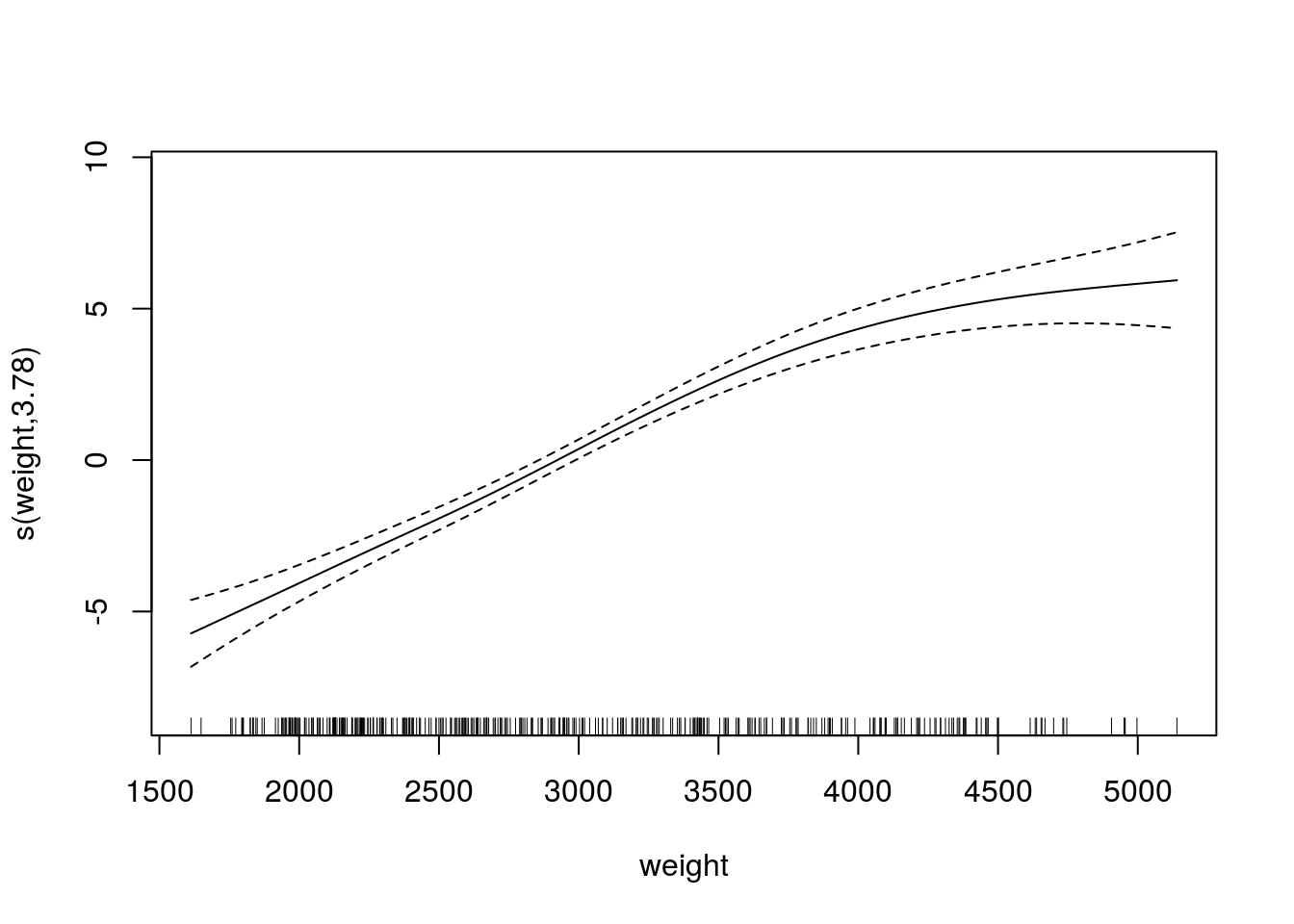We can also look at a 3d graph that includes the linear predictor as well as the two strongest predictors. This is done with the “vis.gam” function. Below is the code

vis.gam(model1)If multiple models are developed. You can compare the GCV values to determine which model is the best. In addition, another way to compare models is with the “AIC” function. In the code below, we will create an additional model that includes “year” compare the GCV scores and calculate the AIC. Below is the code.

model2<-gam(acceleration~s(mpg)+s(displacement)+s(horsepower)+s(weight)+s(year),data=Auto)
summary(model2)
##
## Family: gaussian
##
## Formula:
## acceleration ~ s(mpg) + s(displacement) + s(horsepower) + s(weight) +
##     s(year)
##
## Parametric coefficients:
##             Estimate Std. Error t value Pr(>|t|)
## (Intercept) 15.54133    0.07203   215.8   <2e-16 ***
## ---
## Signif. codes:  0 '***' 0.001 '**' 0.01 '*' 0.05 '.' 0.1 ' ' 1
##
## Approximate significance of smooth terms:
##                   edf Ref.df      F p-value
## s(mpg)          5.578  6.726  2.749  0.0106 *
## s(displacement) 2.251  2.870 13.757 3.5e-08 ***
## s(horsepower)   4.936  6.054 66.476 < 2e-16 ***
## s(weight)       3.444  4.397 34.441 < 2e-16 ***
## s(year)         1.682  2.096  0.543  0.6064
## ---
## Signif. codes:  0 '***' 0.001 '**' 0.01 '*' 0.05 '.' 0.1 ' ' 1
##
## R-sq.(adj) =  0.733   Deviance explained = 74.5%
## GCV = 2.1368  Scale est. = 2.0338    n = 392
#model1 GCV
model1$gcv.ubre ## GCV.Cp ## 2.127589 #model2 GCV model2$gcv.ubre
##   GCV.Cp
## 2.136797

As you can see, the second model has a higher GCV score when compared to the first model. This indicates that the first model is a better choice. This makes sense because in the second model the variable “year” is not significant. To confirm this we will calculate the AIC scores using the AIC function.

AIC(model1,model2)
##              df      AIC
## model1 18.04952 1409.640
## model2 19.89068 1411.156

Again, you can see that model1 s better due to its fewer degrees of freedom and slightly lower AIC score.

Conclusion

Using GAMs is most common for exploring potential relationships in your data. This is stated because they are difficult to interpret and to try and summarize. Therefore, it is normally better to develop a generalized linear model over a GAM due to the difficulty in understanding what the data is trying to tell you when using GAMs.

# Theoretical Distribution and R

This post will explore an example of testing if a dataset fits a specific theoretical distribution. This is a very important aspect of statistical modeling as it allows to understand the normality of the data and the appropriate steps needed to take to prepare for analysis.

In our example, we will use the “Auto” dataset from the “ISLR” package. We will check if the horsepower of the cars in the dataset is normally distributed or not. Below is some initial code to begin the process.

library(ISLR)
library(nortest)
library(fBasics)
data("Auto")

Determining if a dataset is normally distributed is simple in R. This is normally done visually through making a Quantile-Quantile plot (Q-Q plot). It involves using two functions the “qnorm” and the “qqline”. Below is the code for the Q-Q plot

qqnorm(Auto$horsepower)We now need to add the Q-Q line to see how are distribution lines up with the theoretical normal one. Below is the code. Note that we have to repeat the code above in order to get the completed plot. qqnorm(Auto$horsepower)
qqline(Auto$horsepower, distribution = qnorm, probs=c(.25,.75))The “qqline” function needs the data you want to test as well as the distribution and probability. The distribution we wanted is normal and is indicated by the argument “qnorm”. The probs argument means probability. The default values are .25 and .75. The resulting graph indicates that the distribution of “horsepower”, in the “Auto” dataset is not normally distributed. That are particular problems with the lower and upper values. We can confirm our suspicion by running a statistical test. The Anderson-Darling test from the “nortest” package will allow us to test whether our data is normally distributed or not. The code is below ad.test(Auto$horsepower)
##  Anderson-Darling normality test
##
## data:  Auto$horsepower ## A = 12.675, p-value < 2.2e-16 From the results, we can conclude that the data is not normally distributed. This could mean that we may need to use non-parametric tools for statistical analysis. We can further explore our distribution in terms of its skew and kurtosis. Skew measures how far to the left or right the data leans and kurtosis measures how peaked or flat the data is. This is done with the “fBasics” package and the functions “skewness” and “kurtosis”. First we will deal with skewness. Below is the code for calculating skewness. horsepowerSkew<-skewness(Auto$horsepower)
horsepowerSkew
##  1.079019
## attr(,"method")
##  "moment"

We now need to determine if this value of skewness is significantly different from zero. This is done with a simple t-test. We must calculate the t-value before calculating the probability. The standard error of the skew is defined as the square root of six divided by the total number of samples. The code is below

stdErrorHorsepower<-horsepowerSkew/(sqrt(6/length(Auto$horsepower))) stdErrorHorsepower ##  8.721607 ## attr(,"method") ##  "moment" Now we take the standard error of Horsepower and plug this into the “pt” function (t probability) with the degrees of freedom (sample size – 1 = 391) we also put in the number 1 and subtract all of this information. Below is the code 1-pt(stdErrorHorsepower,391) ##  0 ## attr(,"method") ##  "moment" The value zero means that we reject the null hypothesis that the skew is not significantly different form zero and conclude that the skew is different form zero. However, the value of the skew was only 1.1 which is not that non-normal. We will now repeat this process for the kurtosis. The only difference is that instead of taking the square root divided by six we divided by 24 in the example below. horsepowerKurt<-kurtosis(Auto$horsepower)
horsepowerKurt
##  0.6541069
## attr(,"method")
##  "excess"
stdErrorHorsepowerKurt<-horsepowerKurt/(sqrt(24/length(Auto\$horsepower)))
stdErrorHorsepowerKurt
##  2.643542
## attr(,"method")
##  "excess"
1-pt(stdErrorHorsepowerKurt,391)
##  0.004267199
## attr(,"method")
##  "excess"

Again the pvalue is essentially zero, which means that the kurtosis is significantly different from zero. With a value of 2.64 this is not that bad. However, when both skew and kurtosis are non-normally it explains why our overall distributions was not normal either.

Conclusion

This post provided insights into assessing the normality of a dataset. Visually inspection can take place using  Q-Q plots. Statistical inspection can be done through hypothesis testing along with checking skew and kurtosis.# The History and Characteristics of R

R is a programming language and software environment that is used for the development of graphic data products and the computation of many forms of mathematics. The history of R goes back about 20-30 years ago. This post willlook at the history of R as well as the Characteristics of this software.

The History

Ross Ihaka and Robert Gentleman are the developers of R. R is actually based on an older programming language known as S which goes back to the 1970″s. Ihaka and Gentleman develop their own programming language while working together in New Zealand. With the release of R in the early 1990’s, several people joined the project to help to improve it. By 1995, the software had become “open-sourced” which means that anyone can use and modify it for themselves without cost. By 2000, the first version of R (1.0) was released to the public.

Characteristics of R

In many peoples opinion, the best feature of R is the price. Being free, R is by far one of the best softwares for statistical analysis is price is the most important criterion. SPSS and SAS are also great and user-friendlier, however, their price is completely outlandish for most individual researchers. R removes this problem completely

R also has an active community around it that supports its development. For example, people are able to develop packages that provide assistance in running various task in the R software. Naturally, most packages are free as well. The focus on community has enabled R to be run on almost any operating system as well, such as Windows, OSX, or Linux.

R also allows people to make graphs and data products. The graphs are actually very well made. The drawback is understanding the coding necessary to develop these various products. This is discussed more below.

One major drawback that affects the typical computer user is learning the programming language of R. This can be challenging for those who are not techie or able to think abstractly in computer codes. I have never seen a satisfactory way to get around this problem but to crack open a book and practice, practice, practice. With time, the code will start to make sense but it is not a five-minute process for someone who has not studied programming.

Conclusion

Despite the challenges of learning computer programming R is becoming the software of choice for many. The benefits far outweigh the problems for many individuals. Personally, I am looking forward to continuing to develop skills in understanding this dynamic software.

# Population vs Sample

In statistics, one of the most fundamental concepts is the population and sample. A population is all the member from a group. For example, if my population is the United States, I would have to collect data from everyone in the country. This is to say the least, very challenging.

To deal with this, must studies take a sample from the population. A sample is a portion of the population. Continuing our example, instead of collecting data from every in the US I would collect data from several hundred or thousand depending on the research question of my study.

There are several different techniques to sampling that will be covered later.  For now, the most important thing to remember is that your research questions and circumstances of the study influence what steps you take. There is rarely one way to do this.